## How Banks Create Money

### Learning Objectives

• Explain and show how banks create money
• Use the money multiplier formula to calculate how banks create money

## Money Creation by a Single Bank

Banks and money are intertwined. It is not just that most money is in the form of bank accounts. The banking system can literally create money through the process of making loans. Let’s see how.

Start with a hypothetical bank called Singleton Bank. The bank has $10 million in deposits. The T-account balance sheet for Singleton Bank, when it holds all of the deposits in its vaults, is shown in Figure 1. At this stage, Singleton Bank is simply storing money for depositors; it is not using these deposits to make loans, so it cannot pay its depositors interest either.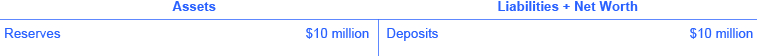Figure 1. Singleton Bank’s Balance Sheet: Receives$10 million in Deposits.

Singleton Bank is required by the Federal Reserve to keep 10% of total deposits, or  $1 million, on reserve to cover withdrawals. It will loan out the remaining$9 million. By loaning out the $9 million and charging interest, it will be able to make interest payments to depositors and earn interest income for Singleton Bank and make interest payments to depositors (for now, we will keep it simple and not put interest income on the balance sheet). Instead of becoming just a storage place for deposits, Singleton Bank can become a financial intermediary between savers and borrowers. This change in business plan alters Singleton Bank’s balance sheet, as shown in Figure 2. Singleton’s assets have changed; it now has$1 million in reserves and a loan to Hank’s Auto Supply of $9 million. The bank still has$10 million in deposits.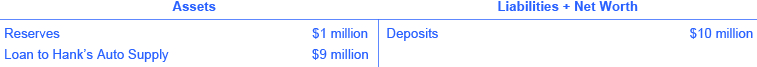Figure 2. Singleton Bank’s Balance Sheet: 10% Reserves, One Round of Loans

Singleton Bank lends $9 million to Hank’s Auto Supply. The bank records this loan by making an entry on the balance sheet to indicate that a loan has been made. This loan is an asset, because it will generate interest income for the bank. Of course, the loan officer is not going to let Hank walk out of the bank with$9 million in cash. The bank issues Hank’s Auto Supply a cashier’s check for the $9 million. Hank deposits the loan in his regular checking account with First National. The deposits at First National rise by$9 million and its reserves also rise by $9 million, as Figure 3 shows. First National must hold 10% of additional deposits as required reserves but is free to loan out the rest.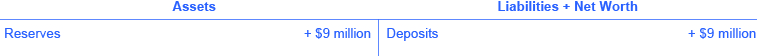Figure 3. First National Bank’s Balance Sheet: Required 10% Reserves Since the loan to Hank was deposited into a demand deposit account (Hank’s checking account), the loan increases the M1 money supply. Making loans that are deposited into a demand deposit account increases the M1 money supply. Remember the definition of M1 includes checkable (demand) deposits, which can be easily used as a medium of exchange to buy goods and services. Notice that the money supply is now$19 million: $10 million in deposits in Singleton bank and$9 million in deposits at First National. Obviously these deposits will be drawn down as Hank’s Auto Supply writes checks to pay its bills, but as long as those checks are deposited in other checking accounts, the effect is the same. The bottom line is that a bank must hold enough money to meet its reserve requirement; the rest the bank loans out, and those loans, when deposited, add to the money supply. In this example so far, bank lending has expanded the money supply by $9 million. Now, First National must hold only 10% as required reserves ($90,000) but can lend out the other 90% ($8.1 million) in a loan to Jack’s Chevy Dealership as shown in Figure 4.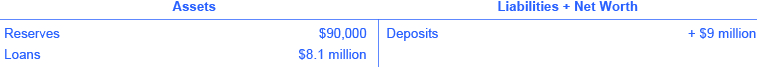Figure 4. First National Balance Sheet If Jack’s deposits the loan in its checking account at Second National, the money supply just increased by an additional$8.1 million, as Figure 5 shows.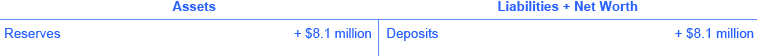Figure 5. Second National Bank’s Balance Sheet

How is this money creation possible? It is possible because there are multiple banks in the financial system, they are required to hold only a fraction of their deposits, and loans end up deposited in other banks, which increases deposits and, in essence, the money supply.

### Watch It

This video explains how banks use deposits and loans to create money.

## The Money Multiplier in a Multi-Bank System

In a system with multiple banks, the initial excess reserve amount that Singleton Bank decided to lend to Hank’s Auto Supply was deposited into First National Bank, which is free to loan out $8.1 million. If all banks loan out their excess reserves, the money supply will expand. In a multi-bank system, the amount of money that the system can create is found by using the money multiplier. The money multiplier tells us by how many times a loan will be “multiplied” through the process of lending out excess reserves, which are deposited in banks as demand deposits. Thus, the money multiplier is the ratio of the change in money supply to the initial change in bank reserves. Fortunately, a formula exists for calculating the total of these many rounds of lending in a banking system. The money multiplier formula is: $\displaystyle\frac{1}{\text{Required Reserve Ratio}}$ ### USING THE MONEY MULTIPLIER FORMULA Using the money multiplier for the example from Singleton Bank above in this text: Step 1. In this example, the reserve requirement is 10% (or 0.10), so the money multiplier is 1 divided by 0.10, which is equal to 10. Step 2. Since Singleton Bank initially has reserves of$10 million, using the formula we can determine the potential amount of new money created by that deposit:

$\displaystyle\text{Total M1 Money Supply Supported by Singleton Bank's Reserves}=\frac{1}{\text{Required Reserve Ratio}}\times\text{Bank Reserves}$

$\displaystyle\text{Total M1 Money Supply Supported by Singleton Bank's Reserves}=\frac{1}{0.10}\times{10}\text{ million}$

$\displaystyle\text{Total M1 Money Supply Supported by Singleton Bank's Reserves}=10\times{10}\text{ million}$

$\displaystyle\text{Total M1 Money Supply}=100\text{ million}$

Step 3. Thus, we can say that, in this example, after all rounds of lending are completed, Singleton Bank’s initial reserves of $10 million will support$100 million in M1 Money Supply.

Step 4. Since initially Singleton Bank started with $10 million in demand deposits (which means that$10 million was already counted in the money supply), we subtract that initial amount from the total.

$\displaystyle\text{Change in M1 Money Supply}=\frac{1}{\text{Required Reserve Ratio}}\times\text{Bank Reserves} - \text{Initial Deposit}$

$\displaystyle\text{Total M1 Money Supply Supported by Singleton Bank's Reserves}=10\times{10}\text{ million} -{10}\text{ million}=90\text{ million}$

So the loan expansion process from Singleton Bank’s Deposit was able to create $90 million in new deposits/money supply. Note that when we talk about changes in the M1 money supply, it makes a difference whether the change in deposits comes from people depositing currency or from the Federal Reserve. If a person takes currency and deposits it into their checking account, their bank holds the required reserves and then lends out the rest, spurring the loan expansion process. The change in the money supply needs to take into account that the currency was already part of M1 and shouldn’t be counted again. Thus, the change in the M1 money supply will be the change in deposits multiplied by the money multiplier minus the decrease in currency held that was deposited in the bank (as shown in this example with Singleton Bank). Let’s look at another example. Suppose Carla deposits$50 in cash into her checking account. The $50 cash was already part of the money supply. If the required reserve ratio is 10%, the money multiplier will be 1/10% = 1/0.10 = 10. Carla’s bank keeps$5 of her deposit as required reserves and loans out the rest. When the loan expansion process in the banking system is complete, the total change in the M1 money supply is 10 times $50 minus the$50 currency that Carla moved from currency to her bank account.

Change in M1 Money Supply = 10 x $50 =$500 – $50 =$450

In the module on monetary policy, we will explain how when the Federal Reserve conducts expansionary monetary policy (ie. purchasing Treasury bonds), the change in deposits comes from outside the financial system. In that case, the change in the money supply will equal the change in deposits times the money multiplier. Since there is no change in currency holdings, we don’t subtract the amount of the initial deposit.

## Cautions about the Money Multiplier

The money multiplier will depend on the proportion of reserves that banks are required to hold by the Federal Reserve Bank. Additionally, a bank can also choose to hold extra reserves. Banks may decide to vary how much they hold in reserves for two reasons: macroeconomic conditions and government rules. When an economy is in recession, banks are likely to hold a higher proportion of reserves because they fear that loans are less likely to be repaid when the economy is slow. The Federal Reserve may also raise or lower the required reserves held by banks as a policy move to affect the quantity of money in an economy, as we will discuss in more depth in the module on monetary policy.

The process of how banks create money shows how the quantity of money in an economy is closely linked to the quantity of lending or credit in the economy. Indeed, all of the money in the economy, except for the original reserves, is a result of bank loans that are re-deposited and loaned out, again, and again.

Finally, the money multiplier depends on people re-depositing the money that they receive in the banking system. If people instead store their cash in safe-deposit boxes or in shoeboxes hidden in their closets, then banks cannot recirculate the money in the form of loans. Indeed, central banks have an incentive to assure that bank deposits are safe because if people worry that they may lose their bank deposits, they may start holding more money in cash, instead of depositing it in banks, and the quantity of loans in an economy will decline. Low-income countries have what economists sometimes refer to as “mattress savings,” or money that people are hiding in their homes because they do not trust banks. When mattress savings in an economy are substantial, banks cannot lend out those funds and the money multiplier cannot operate as effectively. The overall quantity of money and loans in such an economy will decline.

### Watch it

This video explains how money is created and reviews the concepts you just learned about the money multiplier. It also explains a little bit about the Federal Reserve’s involvement in creating new money to buy financial assets, thereby adding reserves to the banking system.

If banks hold the minimum amount of money required by the 10% reserve ratio, then they would lend out 90% of their reserves, and the multiplier would continue to stay around 10. This does not happen in practice, and the multiplier remains closer to 3. When we talk about monetary policy in more depth later, you’ll learn more about other ways that the Federal Reserve may choose to increase the money supply.

### Try It

These questions allow you to get as much practice as you need, as you can click the link at the top of the first question (“Try another version of these questions”) to get a new set of questions. Practice until you feel comfortable doing the questions.

### Glossary

money multiplier:
ratio of total money in the economy divided by the amount of reserves, or the ratio of change in the total money in the economy divided by a change in the amount of reserves; formula for the money multiplier is 1/(required reserve ratio)
required reserve ratio:
percentage of total deposits a bank must hold as reserves